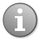19,876 PagesThis is a documentation subpage for Template:Coins.It contains usage information, categories, and other content that is not part of the original template page.

The Coins template shows the amount of coins with a preview image. The amount is formatted with a comma (if the value is greater than 1,000), and with the following colors:

• Red - negative numbers
• Blue - positive numbers
• Green - if the number is zero

## Usage

```{{Coins|Amount=number of coins}}
```

or

```{{Coins|number of coins}}
```

## Examples

The following are some examples:

`{{Coins|Amount=-3000}}`

Produces the following result: -3,000

`{{Coins|Amount=245.50}}`

Produces the following result: 245.5

`{{Coins|Amount=0}}`

Produces the following result: 0